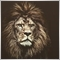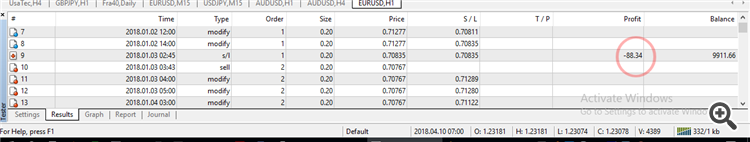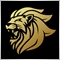# OrderProfit629

Hello,

A trade must be closed if its losses reache -25\$

The probable codes  are :

```for (int pos = 0; pos < OrdersTotal(); pos++){

if ((OrderSelect(pos, SELECT_BY_POS) == TRUE) && (OrderMagicNumber() == magicNumber)) {

if (OrderProfit() >= -25) OrderClose(OrderTicket(), lot, Ask, slipage, 0);

break;

}

}```

is it correct ?Thanks9826

paulselvan:

Hello,

A trade must be closed if its losses reache -25\$

The probable codes  are :

is it correct ?Thanks

Order Profit should be <=-25

`if (OrderProfit() <= -25) OrderClose(OrderTicket(), lot, Ask, slipage, 0);`

Also it is good practice to add some print lines to know whether is there any error in executing these functions.(Also I replaced Ask and lot with OrderClosePrice() and OrderLots())

```if (OrderProfit() <= -25) bool res = OrderClose(OrderTicket(), OrderLots(), OrderClosePrice(), slipage);
if(!res)
Print("Failed to close the Order : ",GetLastError());```

Also I think it is better to filter out the symbol when selecting the order using a for loop.20207

1. Using OrdersTotal directly and/or no Magic number filtering on your OrderSelect loop means your code is incompatible with every EA (including itself on other charts and manual trading.)
Symbol Doesn't equal Ordersymbol when another currency is added to another seperate chart . - MQL4 and MetaTrader 4 - MQL4 programming forum

2. In the presence of multiple orders (one EA multiple charts, multiple EAs, manual trading,) while you are waiting for the current operation (closing, deleting, modifying) to complete, any number of other operations on other orders could have concurrently happened and changed the position indexing:
1. For non-FIFO (US brokers,) (or the EA only opens one order per symbol,) you can simply count down in a position loop, and you won't miss orders. Get in the habit of always counting down.
Loops and Closing or Deleting Orders - MQL4 and MetaTrader 4 - MQL4 programming forum
For FIFO (US brokers,) and you (potentially) process multiple orders per symbol, you must count up and on a successful operation, reprocess all positions (set index to -1 before continuing.)
2. and check OrderSelect in case earlier positions were deleted.
What are Function return values ? How do I use them ? - MQL4 and MetaTrader 4 - MQL4 programming forum
Common Errors in MQL4 Programs and How to Avoid Them - MQL4 Articles
3. and if you (potentially) process multiple orders, must call RefreshRates() after server calls if you want to use the Predefined Variables (Bid/Ask) or OrderClosePrice() instead, on the next order/server call.629

The strategy tester gives -88.34\$ losses more than the limit -25\$Where is the bug in this EA ?

```//+------------------------------------------------------------------+
//|                                               selvan_psar-v2.mq4 |
//|                        Copyright 2018, MetaQuotes Software Corp. |
//|                                             https://www.mql5.com |
//+------------------------------------------------------------------+
#property version   "1.00"
#property strict
//----------
double stopLoss, lot = 0.2;
int  typeTrade, magicNumber = 16844, slipage = 3;
datetime oldTime, newTime;

//----------
int OnInit(){  return(INIT_SUCCEEDED);}
//-------------
void OnDeinit(const int reason){}
//------------
void OnTick(){
Init();

oldTime = Time;
}
else {
newTime = Time;
if (newTime != oldTime){
oldTime = newTime;
}
}
}
//------
for (int pos = 0; pos < OrdersTotal(); pos++)
if (OrderSelect(pos, SELECT_BY_POS) == TRUE) if (OrderMagicNumber() == magicNumber) return(pos);
return(-1);
}
//-----------------------------
int Switch(double now, double old){
int a = -1;
if      ((Low >= now) && (High <= old))   a = OP_BUY;
else if ((High <= now) && (Low >= old))   a = OP_SELL;
return(a);
}
//+------------------------------------------------------------------+
void Init(){
double nowPsar1 = NormalizeDouble(iSAR(NULL, PERIOD_H1, 0.001, 0.2, 0),Digits);
double oldPsar1 = NormalizeDouble(iSAR(NULL, PERIOD_H1, 0.001, 0.2, 1),Digits);
double switch1 = Switch(nowPsar1, oldPsar1);

double nowPsar2 = NormalizeDouble(iSAR(NULL, PERIOD_H1, 0.005, 0.2, 0),Digits);
double oldPsar2 = NormalizeDouble(iSAR(NULL, PERIOD_H1, 0.005, 0.2, 1),Digits);
double switch2 = Switch(nowPsar2, oldPsar2);

bool buy = (Low >= iSAR(NULL, PERIOD_H4, 0.02, 0.2, 0));
bool sell = (High <= iSAR(NULL, PERIOD_H4, 0.02, 0.2, 0));

stopLoss = nowPsar2;

if ((switch2 == OP_SELL) && (nowPsar1 <= oldPsar1) && sell) typeTrade = OP_SELL;
if ((switch1 == OP_SELL) && (nowPsar2 <= oldPsar2) && sell) typeTrade = OP_SELL;
if ((switch2 == OP_SELL) && (switch1 == OP_SELL) && sell) typeTrade = OP_SELL;
}
//-----------
if (type != -1)
OrderSend(NULL, type, lot, Ask*(type == OP_BUY) + Bid*(type == OP_SELL), slipage,0, 0, NULL, magicNumber, 0, clrBlue*(type == OP_BUY) + clrRed*(type == OP_SELL));
}
//-----------
void MoveSL(int type){

if (OrderProfit()<= -25) OrderClose(OrderTicket(), lot, Ask*(type == OP_BUY) + Bid*(type == OP_SELL), slipage, 0);
else
OrderModify(OrderTicket() , OrderOpenPrice(), stopLoss, 0, 0, 0);

}
```9826

paulselvan:

The strategy tester gives -88.34\$ losses more than the limit -25\$

Where is the bug in this EA ?

Did you try changing the closing price to OrderClosePrice(),
Also let me know what is the error that you get , by using GetLastError()Moderator
12666

Because it will only call MoveSL here:

```      newTime = Time;
if (newTime != oldTime){
oldTime = newTime;
}```

Which means it will only check and close the position every time a new bar opens, so in between new bars the loss can drop to any value.

And then when it is dropped to -\$80 and a new bar opens it will of course close the position at a much higher loss.

The modify also times reveal the issue.629

You're right prices drop between new candles  checking.

I modify  the program o run it for every tick instead of every new candle but the bug is still here.

``` else {
//newTime = Time;
//if (newTime != oldTime){
//   oldTime = newTime;
//}
}```

-------------------

I suppose the bug comes from this if..else condition where only else is excuted  :

```void MoveSL(int type){

if (OrderProfit()<= -25) OrderClose(OrderTicket(), lot, Ask*(type == OP_BUY) + Bid*(type == OP_SELL), slipage, 0);
else OrderModify(OrderTicket() , OrderOpenPrice(), stopLoss, 0, 0, 0);

}```

Why only OrderModify() is always exceuted , and OrderClose() is never .629

Lakshan Perera:
Did you try changing the closing price to OrderClosePrice(),
Also let me know what is the error that you get , by using GetLastError()

I understand the use of checking errors

But when in EA strategy tester how to display GetLastError() .

Is Alert() work while in tester ?9826

paulselvan:

I understand the use of checking errors

But when in EA strategy tester how to display GetLastError() .

Is Alert() work while in tester ?

Alert won't pop up a message in strategy tester but it will write on the strategy tester journal629

Lakshan Perera:
Alert won't pop up a message in strategy tester but it will write on the strategy tester journal

The strategy tester journal messages   are :  OrderClose()  error 1389826

paulselvan:

The strategy tester journal messages   are :  OrderClose()  error 138

If it is a buy order it should be closed at Bid, if it is a sell it should be closed at Ask price

`if (OrderProfit()<= -25) OrderClose(OrderTicket(), lot, Bid*(type == OP_BUY) + Ask*(type == OP_SELL), slipage, 0);`

Or just use OrderClosePrice () (it will close the trade at the appropriate price for each order type),

`if (OrderProfit() <= -25) bool res = OrderClose(OrderTicket(), OrderLots(), OrderClosePrice(), slipage);`
Also try increasing the slippage.